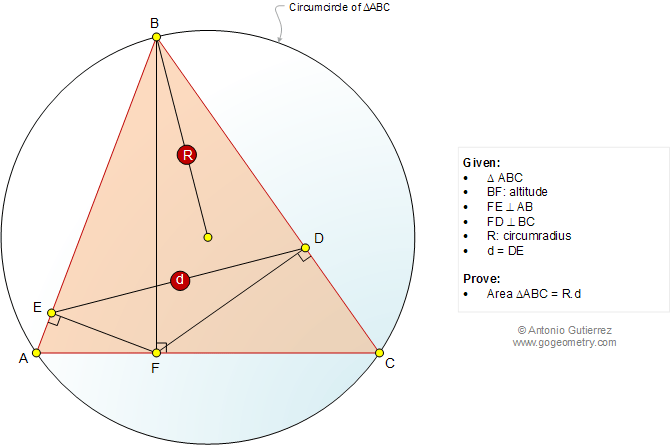# Infographic Geometry Problem 1055: Triangle, Altitude, Perpendicular, Area, Circumradius, Circle. Level: High School, SAT Prep, College, Mathematics Education

< PREVIOUS PROBLEM  |  NEXT PROBLEM >

 In a triangle ABC, R is the circumradius and BF is the altitude (see the figure below). FE and FD are perpendicular to AB and BC at E and D, respectively. If DE = d, prove that area of triangle ABC = R.dHome | Search | Geometry | Problems | All Problems | Open Problems | Visual Index | 10 Problems | 1051-1060 | Triangle | Area | Triangle Area | Altitude | Perpendicular lines | Circle | Circumradius | Email | by Antonio Gutierrez Add or view a solution to the problem 1055 Last updated: Dec 2, 2014